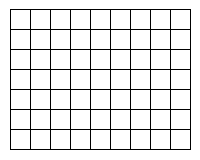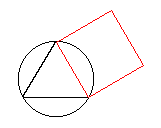Cleave Books
Puzzle Trio 4

10. A pandigital sum (for addition, subtraction, multiplication or division) is one which uses all of the digits 1 to 9 once and once only.
For example -
 128 + 439 = 567 is a pandigital addition sum.
Find another one.

 11 How many distinct squares (not necessarily different in size) can be traced out following only the lines of the grid drawn on the right?12A very old problem is that of "squaring the circle". This means finding a way of constructing a square which is equal in area to a given circle. Many have attempted to solve this problem but were doomed to failure since it has been proved to be impossible. One method that was suggested was as follows - Draw the largest possible equilateral triangle inside the circle. Then draw a square which has one edge in common with the triangle and this square should have the same area as the circle. It is close, but not exact. What is the difference in area between the square and the circle, expressed as a percentage of the area of the circle?